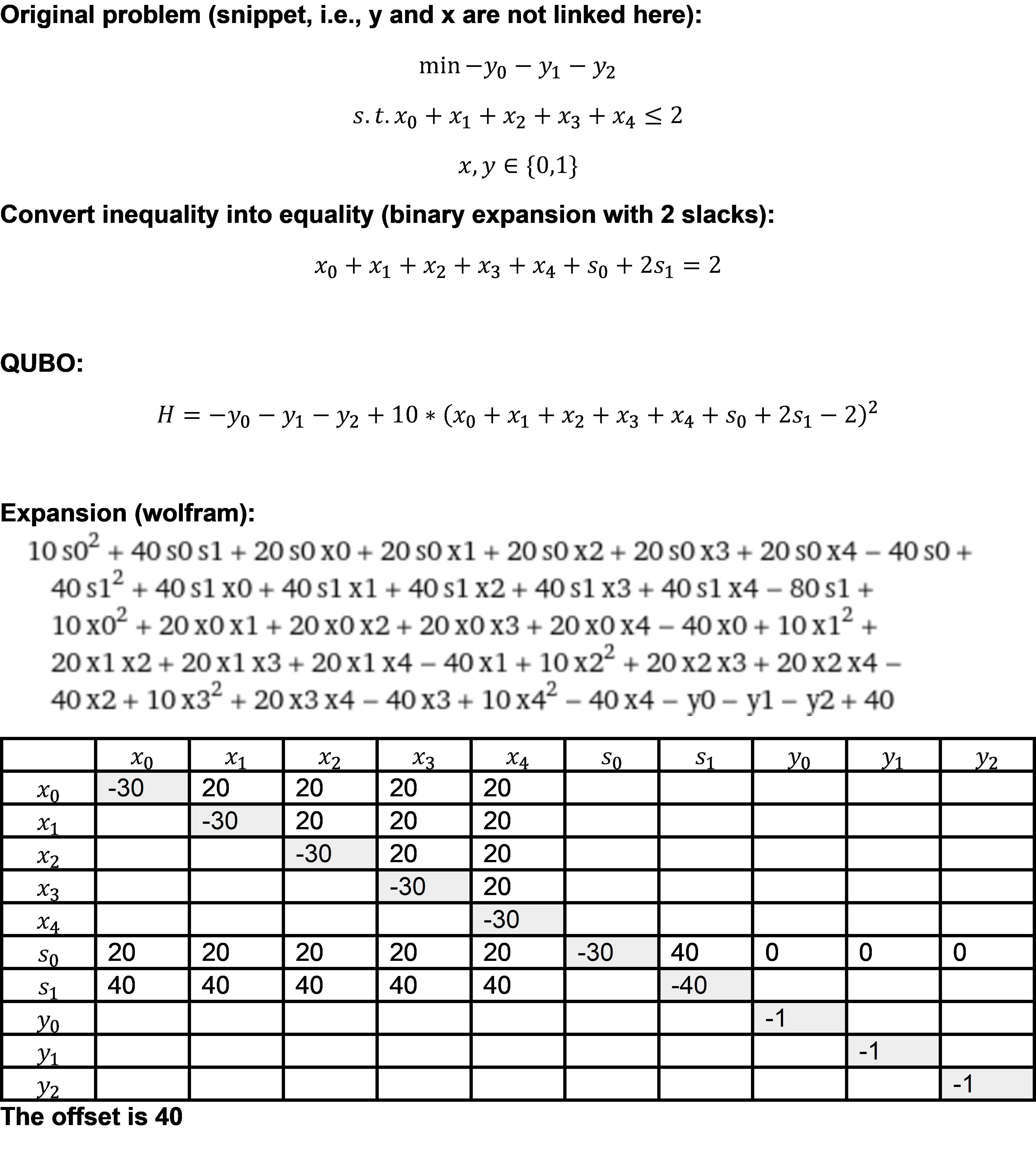# BQM - problem adding an inequality constraint

Hello,

I think I may have stumbled upon a bug with bqm.add_linear_inequality_constraint() or (hopefully) I have misunderstood the intended use of this method.

If I understand correctly bqm.add_linear_inequality_constraint() will convert the inequality using a binary expansion. The number of slacks seems to be consistent with that, however, the coefficients do not seem right. See my case below.

Note: if the RHS of the inequality is 3 then coefficients are correct. If RHS is 4, 3 slacks will be added, however, the coefficient of the last slack is wrong.Implementation:

M stands for the RHS of the inequality

`# Define modelx = [i for i in cuts]y = [j for j in solutions]bqm = dimod.BinaryQuadraticModel('BINARY')# Objective functionfor i in range(len(cuts)):  bqm.add_variable(x[i], 0)for j in range(len(solutions)):  bqm.add_variable(y[j], -1)# Add constraintsc3 = [(x[i], 1) for i in range(len(cuts))]bqm.add_linear_inequality_constraint(c3,                                   constant=-M,                                   lagrange_multiplier=10,                                   label='cut_maximum')`

Results:

pprint(dict(bqm.linear))

`{'slack_cut_maximum_0': -30.0,'slack_cut_maximum_1': -30.0,'x0': -30.0,'x1': -30.0,'x2': -30.0,'x3': -30.0,'x4': -30.0,'y0': -1.0,'y1': -1.0,'y2': -1.0}`

`{('slack_cut_maximum_0', 'x0'): 20.0,('slack_cut_maximum_0', 'x1'): 20.0,('slack_cut_maximum_0', 'x2'): 20.0,('slack_cut_maximum_0', 'x3'): 20.0,('slack_cut_maximum_0', 'x4'): 20.0,('slack_cut_maximum_1', 'slack_cut_maximum_0'): 20.0,('slack_cut_maximum_1', 'x0'): 20.0,('slack_cut_maximum_1', 'x1'): 20.0,('slack_cut_maximum_1', 'x2'): 20.0,('slack_cut_maximum_1', 'x3'): 20.0,('slack_cut_maximum_1', 'x4'): 20.0,('x1', 'x0'): 20.0,('x2', 'x0'): 20.0,('x2', 'x1'): 20.0,('x3', 'x0'): 20.0,('x3', 'x1'): 20.0,('x3', 'x2'): 20.0,('x4', 'x0'): 20.0,('x4', 'x1'): 20.0,('x4', 'x2'): 20.0,('x4', 'x3'): 20.0}`
`pprint(bqm.offset)40.0`

You may observe:

1) The linear terms are correct except for 'slack_cut_maximum_1' which should be -40

2) The quadratic terms for variables are correct except for the slack interactions

3) The offset is correct

• I think I have an answer to my problem after inspecting the source code more carefully (dimod/binary/binary_quadratic_model.py).

Using 2 slack variables(s and s) one can represent the integers 0, 1, 2, 3 if the coefficients are 1 and 2 respectively. In the example above, the maximum integer that must be represented is 2, i.e., the coefficients are 1 and 1 respectively.

The original constraint x+x+x+x+x <= 2 becomes x+x+x+x+x + s+[s1]  = 2

(x+x+x+x+x + s+[s1]  - 2)^2 is positive iff x+x+x+x+x > 2

-------

I was assuming that constraint was represented as x+x+x+x+x + 1*s+2*s =2.

I would limit the maximum integers that the slacks with their original coefficients can represent as follows:

x+x+x+x+x + {1*s+2*s - (2^2-1-2)} =2.

I couldn't find any documentation on this method, maybe it's useful to add it?

• Hello,

I passed along your message to the development team.

There are a number of ways to approach constraint formulation, and we encourage people to explore the different implementations and observe the results produced.

Due to the system being a physical machine, there are some implementations that prove to render more reliable results than others.

If you feel that you have a useful implementation that you would like to share with the community, we strongly recommend you to submit a pull request to the GitHub repository.
This way, your contributions will become part of our official libraries!

In some instances it might also make sense to make a feature request, if some functionality seems to be missing.

Even just posting here helps other users understand how one might approach different problems, which is really great!
Thank you for your active participation in the community and for reaching out to share your observations.

• Hi David,

Thanks for the response. I will do some more testing and report my findings later on. It looks like constraint handling is a very tricky topic so it's indeed useful to share experiences.